# AP Board 7th Class Maths Solutions Chapter 1 Integers Ex 3

AP State Syllabus AP Board 7th Class Maths Solutions Chapter 1 Integers Ex 3 Textbook Questions and Answers.

## AP State Syllabus 7th Class Maths Solutions 1st Lesson Integers Exercise 3Question 1.
Represent the following subtractions on the number line.
(i) 7 – 2
(ii) 8 – ( – 7)
(iii) 3 – 7
(iv) 1 5 – 14
(v) 5 – ( – 8)
(vi) ( – 2) – ( – 1)
Solution:
(i) 7 – 2 = 5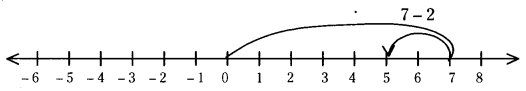(ii) 8 – ( – 7) = 15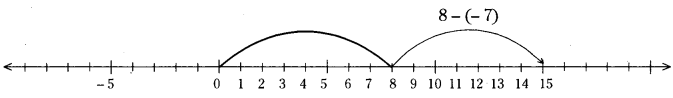(iii) 3 – 7 = -4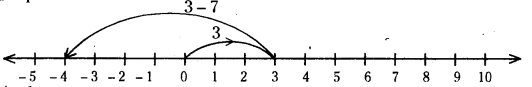(iv) 1 5 – 14 = 1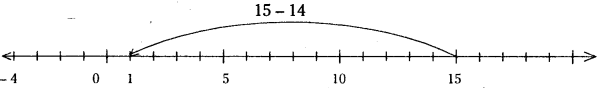(v) 5 – ( – 8) = 13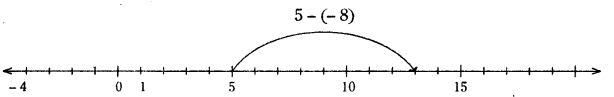(vi) ( – 2) – ( – 1) = -2 + 1 = -1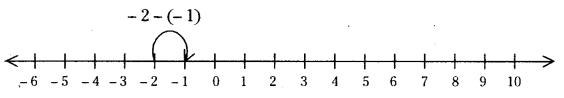Question 2.
Solve the following.
(i) 17 – ( – 14)
(ii) 13 – ( – 8)
(iii) 19 – ( – 5)
(iv) 15 – 28
(v) 25 – 33
(vi) 80 – ( – 50)
(vii) 150 – 75
(viii) 32 – ( – 18)
Solution:
(i) 17 – ( – 14) = 31
(ii) 13 – ( – 8) = 21
(iii) 19 – ( – 5) = 24
(iv) 15 – 28 = -13
(v) 25 – 33 = -8
(vi) 80 – ( – 50) = 130
(vii) 150 – 75 = 75
(viii) 32 – ( – 18) = 50Question 3.
Express ‘ – 6’ as the difference between a negative integer and a whole number.
Solution:
– 6=( – 4) – (2) ( – 5) – (1)=( – 2) – (4)